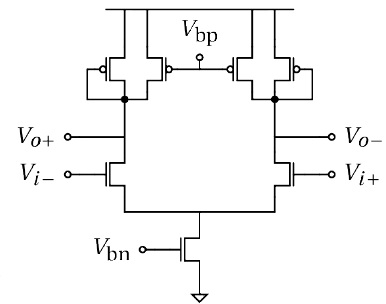# calculation the delay time of symmetric load delay cell

1. ## calculation the delay time of symmetric load delay cell

hi
I want to calculate delay time for this cell with analytic equation and software, but I don't know how I do this
I would be glad if anyone help me to do this2. ## Re: calculation the delay time of symmetric load delay cell•

3. ## Re: calculation the delay time of symmetric load delay cell

dont have any idea??•

4. ## Re: calculation the delay time of symmetric load delay cell

:(:(:(:(:(•

5. ## Re: calculation the delay time of symmetric load delay cell

Delay time by the definition is the time between when the input crosses its 50% of final value and when the output crosses its 50% of final value. In order to calculate (or simulate) the delay time, a step input should be put in the input and calculate (or measure) the time between two mentioned crossings.
The circuit is generally nonlinear, so you can not use half circuit approach. In order to calculate precise delay, it's necessary to observe transistors in different moments of transaction and write correct formulas considering transistors' working regions. It could be a tough job, however there may be some approximate solutions.

1 members found this post helpful.6. ## Re: calculation the delay time of symmetric load delay cellOriginally Posted by salehkhanDelay time by the definition is the time between when the input crosses its 50% of final value and when the output crosses its 50% of final value. In order to calculate (or simulate) the delay time, a step input should be put in the input and calculate (or measure) the time between two mentioned crossings.
The circuit is generally nonlinear, so you can not use half circuit approach. In order to calculate precise delay, it's necessary to observe transistors in different moments of transaction and write correct formulas considering transistors' working regions. It could be a tough job, however there may be some approximate solutions.
I want to calculate the delay time with approximate solutions but I don't know what approximations can be consider
do you have any idea to start and drive the delay equation?, or a good link for me?7. ## Re: calculation the delay time of symmetric load delay cell

Start by making some simplifying assumptions. Your circuit can be modeled as the following picture:Assume that for a long time before t=0:
Vin+=0 and Vin-=Vdd
and after t=0:
Vin+=Vdd and Vin-=0
Then continue writing equations and determine what happens for (Vout2-Vout1).
I can't help further because doing a complete analyze is in the scope of a research paper!

1 members found this post helpful.8. ## Re: calculation the delay time of symmetric load delay cellOriginally Posted by salehkhanStart by making some simplifying assumptions. Your circuit can be modeled as the following picture:Assume that for a long time before t=0:
Vin+=0 and Vin-=Vdd
and after t=0:
Vin+=Vdd and Vin-=0
Then continue writing equations and determine what happens for (Vout2-Vout1).
I can't help further because doing a complete analyze is in the scope of a research paper!
Thanks dear salehkhan for your helps
yes its correct and I dont want complete analyzes, but which simplifying assumptions leads to this circuit?
do you have a link for a research paper related to my work?•

9. ## Re: calculation the delay time of symmetric load delay cell

The resistors are not necessarily linear, actually, I meant that solving the above circuit can help you have an idea about your own circuit.

Becoming curious I found this paper:
"Low-Jitter Process-Independent DLL and PLL Based on Self-Biased Techniques"
by John G. Maneatis

That's all you wantAs you know, I can't put it here.--[[ ]]--